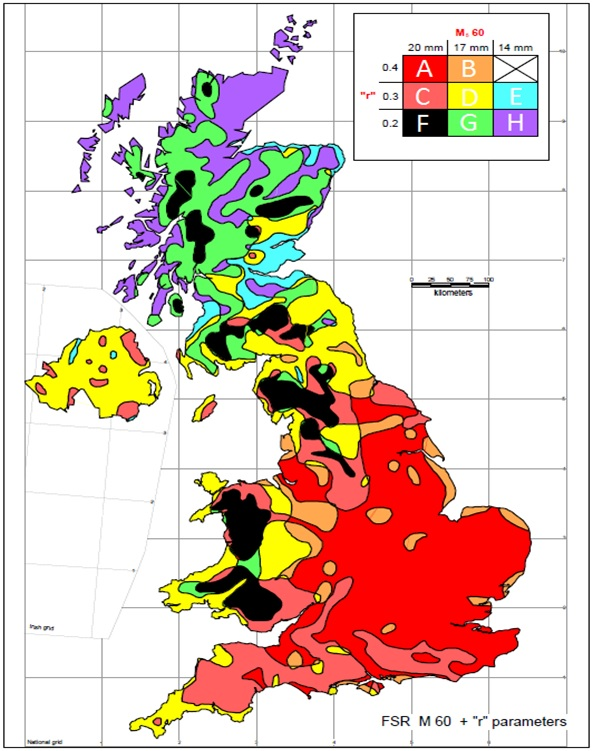# Rainfall Zone

## Rainfall Zone

The UK is divided into 8 different zones describing their rainfall characteristics. Each zone has different factors for rainfall intensity and the intensity of storm events. A map showing the different zones is given below.### Related Spreadsheets from CivilWeb;

##### Colebrook White Pipe Design Spreadsheet

This spreadsheet uses the Colebrook-White and Darcy-Weisbach formulas to calculate the flow conditions in a circular pipe acting under gravity.

### Find Out More

#### Manning Pipe Design Spreadsheet

This spreadsheet uses the Manning formula to calculate the flow conditions in a circular pipe acting under gravity only.

#### Manning Open Channel Design Spreadsheet

This spreadsheet uses the Manning formula to calculate the flow conditions in an open channel acting under gravity only.

##### Runoff Calculator Spreadsheet

This spreadsheet calculates the design runoff flow for a site in accordance with the Flood Estimation Handbook.

#### Linear Drainage Design Spreadsheet

This spreadsheet calculates the maximum flow from a specified linear drainage channel and checks whether the channel is sufficient for the specified site and storm conditions.

#### Attenuation Design Spreadsheet

This spreadsheet calculates the requirements for a attenuation system and assists the user to design a suitable system.

### Soakaway Design Spreadsheet

This spreadsheet calculates the requirements for a soakaway system and assists the user to design a suitable system.

### Full Drainage Design Suite

Full drainage design suite (50% Discount) including 7 spreadsheets;

• Colebrook White Pipe Design
• Manning Pipe Design
• Manning Open Channel Design
• Linear Drainage Design
• Runoff Calculator
• Attenuation Design
• Soakaway Design